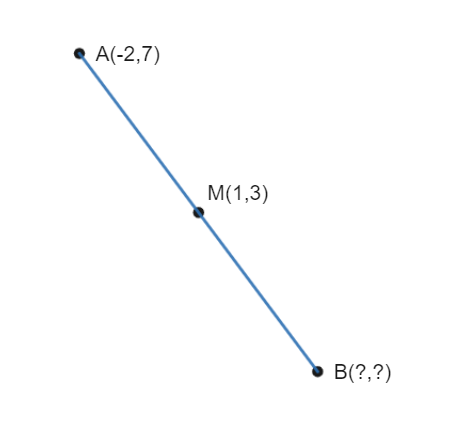Still have math questions?

Geometry
Question

The midpoint of $$\mathrm{AB}$$ is $$\mathrm{M}=(1,3)$$. One endpoint is $$\mathrm{A}=(-2,7)$$. Find the coordinate of the other endpoint, $$\mathrm{B}$$.$$\mathrm{B}(4,-1)$$